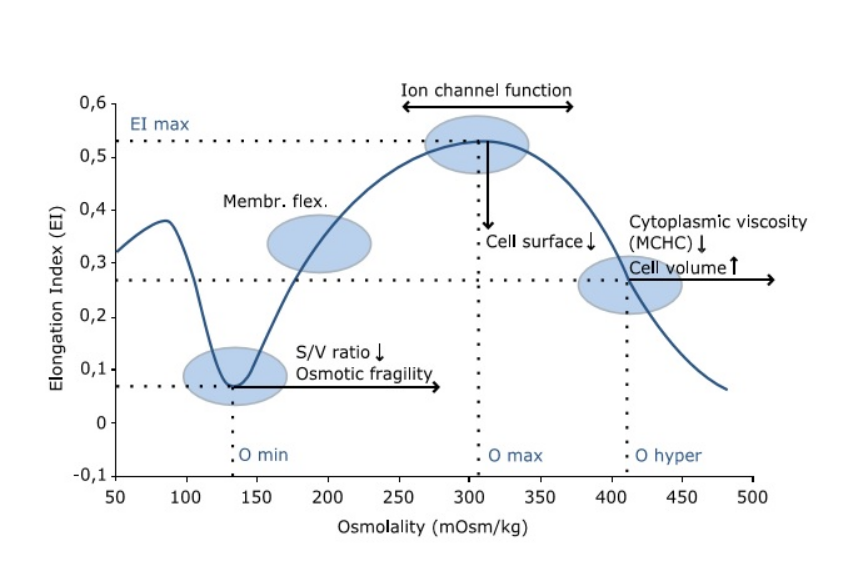## Overview

The Osmoscan measures the deformability under an osmotic gradient. Under shear stress, RBCs can become elongated, and this is quantified by the elongation index (EI). EImax denotes the maximum EI, which is a measure of RBC deformability (or, conversely, fragility).

The 0 min is the osmolality at minimum EI, which is an index of the surface-to-volume ratio of the RBC. The 0 0 min corresponds to the osmolality at 50 % lysis in standard oF testing.

0 hyper is the osmolality at EImax/2, which is an index of RBC hydration (which is important in RBC health).For example Increased 0 min (decreased surface-to-volume ratio), decreased EImax (decreased deformability), and decreased 0 hyper (RBC dehydration).Source: Lorrca.com